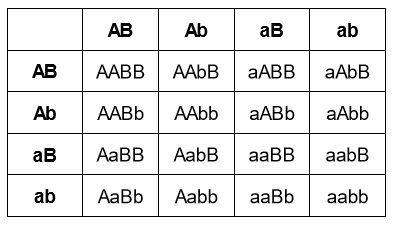# IMAT 2012 Q48 [Genetic Crossing]

In a set of genetic crosses the offspring produced showed the same phenotype ratio of 9:3:3:1.

Which of the following statements could be true?

1. two genes each with two alleles were studied
2. all parents were heterozygous
3. some offspring had a phenotype different to the parents
4. some offspring had a phenotype the same as the parents

A. 1, 2, 3 and 4
B. 1 and 2 only
C. 3 and 4 only
D. 2 and 3 only
E. 1 only

The answer to this question is A, all statements, 1-4, are true.

The rationale behind this answer is the major clue that was given in this question. There was a mention of the phenotype ratio of 9:3:3:1, which should prompt you to recognize that this is the phenotype ratio of a dihybrid cross.

Let us then analyze why each of the numbers correctly describe a dihybrid cross.

"Two genes each with two alleles were studied."

• A dihybrid cross is a cross between two organisms with 2 different traits. In other words, this cross is concerned with 2 genes, where each gene controls 1 trait. Since a gene is made up of 2 alleles, this statement correctly describes the main essence of a dihybrid cross.

"All parents were heterozygous."

• Keep in mind that the phenotypic ratio that was given in this question was 9:3:3:1. If you were to construct a punnett square to come up with that specific ratio, you would need both parents to be heterozygous, where both of the genes they carry are heterozygous. An example would be if both parents had the genotype AaBb.

"Some offspring had a phenotype different to the parents." & “Some offspring had a phenotype the same as the parents”.

• For the sake of example, let us say that both parents had a genotype of AaBb.
• If you were to draw a punnett square to visualize the dihybrid cross, it would look like this:• As you can see in the image, if you were to tally the different possible combinations of A, a, B, and b, you will come up with the phenotypic ratio of 9:3:3:1. You will also observe that the genotype AaBb happens 4 times, which supports statement #3 given in the choices, where it is possible for some offspring to have the same genotype as their parents. At the same time, you can also see other genotypes that are not AaBb, which supports statement #4.

Thus, the answer to this question is A, where all statements correctly describe the dihybrid cross.